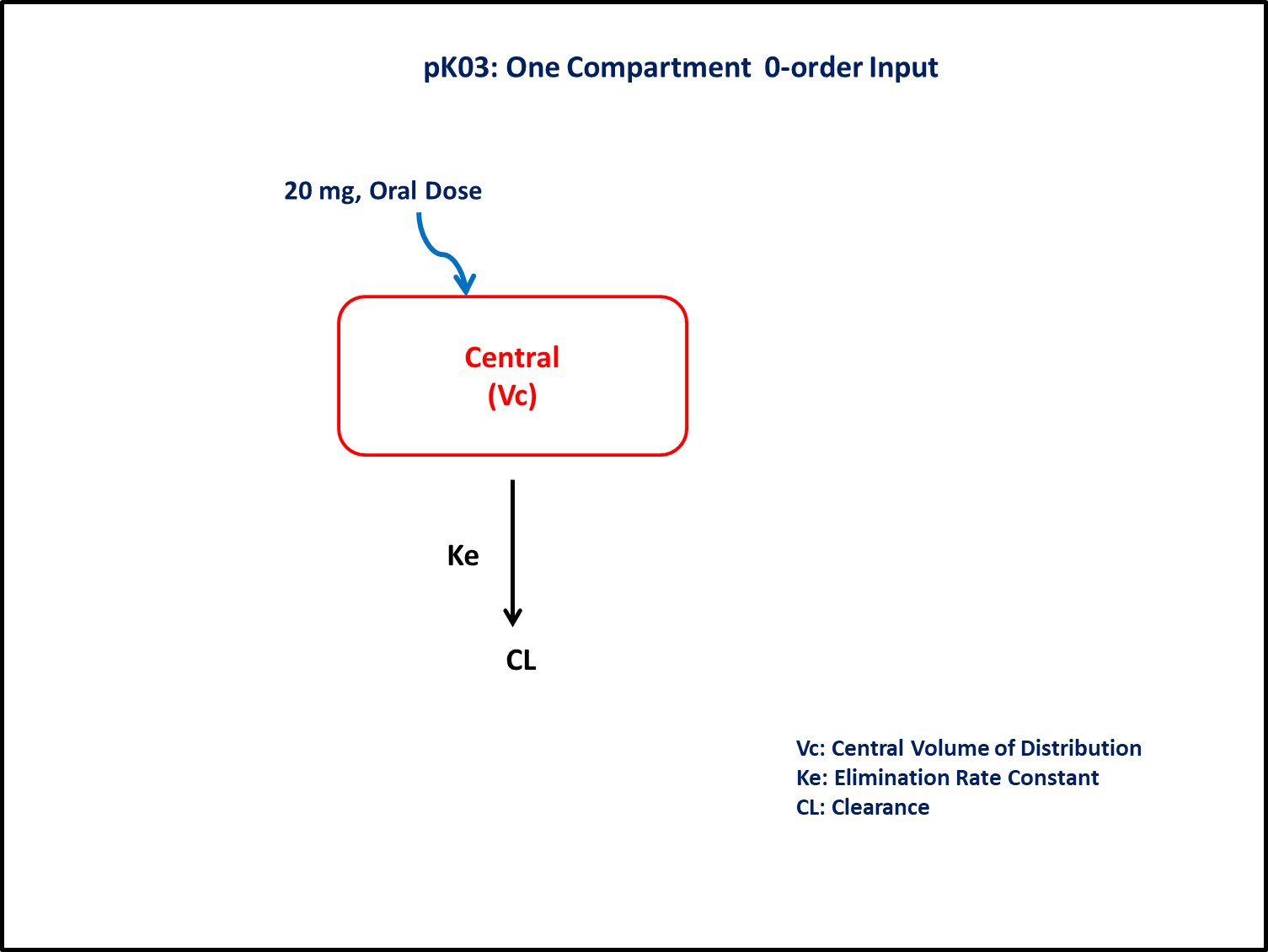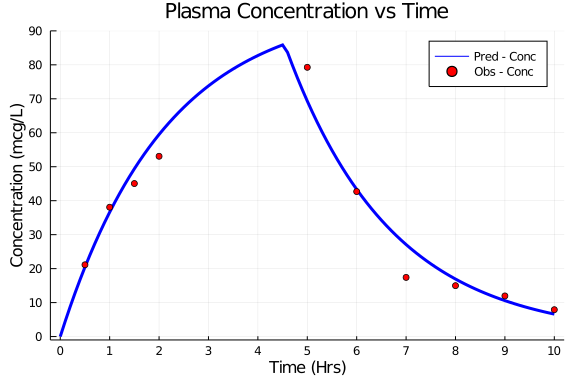# Exercise PK03 - One Compartment 1ˢᵗ- and 0-order input

### Background

Following info:

• Structural model - One compartment linear elimination with zero-order absorption

• Route of administration - Oral

• Dosage Regimen - 20 mg Oral

• Number of Subjects - 1In this model, collection of plasma concentration data, will help you to derive/estimate the parameters: Clearance, Volume of Distribution, Duration of zero-order input.

### Objectives

In this tutorial, you will learn how to build one compartment model for zero-order input and simulate the model for a single subject.

### Libraries

call the "necessary" libraries to get started.

using Pumas
using Plots
using CSV
using StatsPlots
using Random


### Model

In this one compartment model, we administer dose in Central compartment as a zero-order input and estimate the rate of input.

pk_03        = @model begin
@param begin
tvcl     ∈ RealDomain(lower=0)
tvvc     ∈ RealDomain(lower=0)
tvTabs   ∈ RealDomain(lower=0)
Ω        ∈ PDiagDomain(3)
σ²_prop  ∈ RealDomain(lower=0)
end

@random begin
η        ~ MvNormal(Ω)
end

@pre begin
Cl       = tvcl * exp(η)
Vc       = tvvc * exp(η)
duration = (Central = tvTabs * exp(η),)
end

@dynamics begin
Central' =  - (Cl/Vc)*Central
end

@derived begin
cp       = @. 1000*(Central/Vc)
dv       ~ @. Normal(cp, sqrt(cp^2*σ²_prop))
end
end

PumasModel
Parameters: tvcl, tvvc, tvTabs, Ω, σ²_prop
Random effects: η
Covariates:
Dynamical variables: Central
Derived: cp, dv
Observed: cp, dv


### Parameters

• Cl - Clearance (L/hr)

• Vc - Volume of Central Compartment (L)

• Tabs - Assumed duration of zero-order input (hrs)

• Ω - Between Subject Variability

• σ - Residual error

param = ( tvcl     = 45.12,
tvvc     = 96,
tvTabs   = 4.54,
Ω        = Diagonal([0.0,0.0,0.0]),
σ²_prop  = 0.015)

(tvcl = 45.12, tvvc = 96, tvTabs = 4.54, Ω = [0.0 0.0 0.0; 0.0 0.0 0.0; 0.0
0.0 0.0], σ²_prop = 0.015)


### Dosage Regimen

Single 20 mg or 20000μg Oral dose given to a subject.

Note:- In this the dose administered is on mg and conc are in μg/L, hence a scaling factor of 1000 is used in the @derived block in the model.

ev1   = DosageRegimen(20,rate=-2)
sub1  = Subject(id=1,events=ev1)

Subject
ID: 1
Events: 2


### Simulation

Lets simulate for plasma concentration with the specific observation time points after oral administration.

Random.seed!(123)
sim_sub1 = simobs(pk_03, sub1, param, obstimes=0:0.1:10);


### Dataframe & Plot

Create a dataframe of simulated data.

df1    = DataFrame(sim_sub1)
df1_dv = filter(x -> x.time in [0.5,1,1.5,2,3,4,5,6,7,8,9,10], df1)

#Plot the simulated plasma concentration data.

@df df1 plot(:time, :cp,
label= "Pred - Conc",  xlabel="Time (Hrs)", ylabel="Concentration (mcg/L)",
color=[:blue],linewidth=3, title="Plasma Concentration vs Time",
xlims=(-0.2,10.2), xticks=[0,1,2,3,4,5,6,7,8,9,10], yticks=[0,10,20,30,40,50,60,70,80,90], ylims=(-1,90))
@df df1_dv scatter!(:time, :dv, label="Obs - Conc", color=[:red])